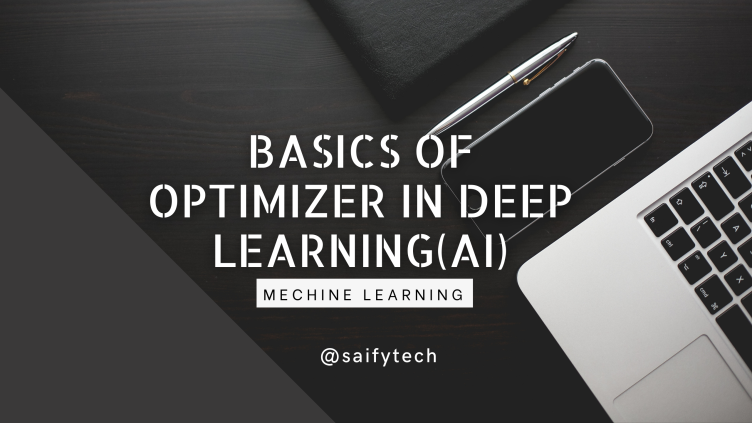# BASICS OF OPTIMIZER IN DEEP LEARNING(AI)

Optimizer as the name suggest to optimize something.It is used to optimize and help to attain models efficiency and accuracy of prediction.It is the component in deep learning which closely works with loss function to improve model.Optimizer uses learning rate to increase or decrease weights and bias for model improvement.

# How optimizer works?

According to universal approximation theorem anything in this world can be expressed in terms of function . To achieve that we use deep learning.In deep learning till now we have discussed about data, architecture and loss function.As we have seen in the previous post that loss function provide us direction and gradient to improve our model.If you haven't read the post kindly click here.After getting gradients and direction from loss function from differentiation, then we take step to change weights and bias of our model.

Firstly we will defined terms which is going to be used in following post to make you understand optimizer.

GRADIENT:-It is numerical number which is created through differentiation of loss function.

DIRECTION:-The nature of that numerical number which is created through differentiation is the direction in which we have move.Let say we get differentiation as -7.0. (-)this sign indicates us that in which direction we have to go.

STEPS:-How much steps you have taken to reach local minimum of your model.

LOCAL MINIMUM:-It is highest efficiency our model can achieve through this process.

Learning Rate:-It is numerical value which shows how much distance you have to cover in each steps.

# Technical Understanding:-

Let say you have a model whose gradient plot is of above shape.According to Universal Approximation theorem if loss function values are less than model is more efficient. Your model efficiency is inversely proportional to loss function values.As loss function values decreases your model's efficiency will increase.So we take steps to decrease our loss function values.To decrease our loss function values we change weights and biases of our model by subtracting gradient into learning rate from weights and biases.

So through this our weights will change and our model efficiency change.

Perceptual Understanding:-

We have many default optimizer presents to use in deep learning which are stochastic gradient descent,ADAGRAD,ADAM etc.In all of above we provide learning rate to achieve efficiency of model.

CONCLUSION:-In this post we have explore basics of optimizer in which we have seen use of learning rate,gradient, direction.We try to understand in both technical and conceptual manner of optimizer in deep learning.